Documentation

## Estimate Nonlinear Grey-Box Models

### Specifying the Nonlinear Grey-Box Model Structure

You must represent your system as a set of first-order nonlinear difference or differential equations:

`$\begin{array}{l}{x}^{†}\left(t\right)=F\left(t,x\left(t\right),u\left(t\right),par1,par2,...,parN\right)\\ y\left(t\right)=H\left(t,x\left(t\right),u\left(t\right),par1,par2,...,parN\right)+e\left(t\right)\\ x\left(0\right)=x0\end{array}$`

where ${x}^{†}\left(t\right)=dx\left(t\right)}{dt}$ for continuous-time representation and ${x}^{†}\left(t\right)=x\left(t+{T}_{s}\right)$ for discrete-time representation with Ts as the sample time. F and H are arbitrary linear or nonlinear functions with Nx and Ny components, respectively. Nx is the number of states and Ny is the number of outputs.

After you establish the equations for your system, create a function or MEX-file. MEX-files, which can be created in C or Fortran, are dynamically linked subroutines that can be loaded and executed by the MATLAB®. For more information about MEX-files, see C MEX File Applications (MATLAB). This file is called an ODE file or a model file.

The purpose of the model file is to return the state derivatives and model outputs as a function of time, states, inputs, and model parameters, as follows:

`[dx,y] = MODFILENAME(t,x,u,p1,p2, ...,pN,FileArgument)`

### Tip

The template file for writing the C MEX-file, `IDNLGREY_MODEL_TEMPLATE.c`, is located in `matlab/toolbox/ident/nlident`.

The output variables are:

• `dx` — Represents the right side(s) of the state-space equation(s). A column vector with Nx entries. For static models, `dx=[]`.

For discrete-time models. `dx` is the value of the states at the next time step `x(t+Ts)`.

For continuous-time models. `dx` is the state derivatives at time t, or $\frac{dx}{dt}$.

• `y` — Represents the right side(s) of the output equation(s). A column vector with Ny entries.

The file inputs are:

• `t` — Current time.

• `x` — State vector at time `t`. For static models, equals `[]`.

• `u` — Input vector at time `t`. For time-series models, equals `[]`.

• `p1,p2, ...,pN` — Parameters, which can be real scalars, column vectors or two-dimensional matrices. `N` is the number of parameter objects. For scalar parameters, `N` is the total number of parameter elements.

• `FileArgument` — Contains auxiliary variables that might be required for updating the constants in the state equations.

### Tip

After creating a model file, call it directly from the MATLAB software with reasonable inputs and verify the output values. Also check that for the expected input and parameter value ranges, the model output and derivatives remain finite.

For an example of creating grey-box model files and `idnlgrey` model object, see Creating idnlgrey Model Files.

For examples of code files and MEX-files that specify model structure, see the `toolbox/ident/iddemos/examples` folder. For example, the model of a DC motor is described in files `dcmotor_m` and `dcmotor_c`.

### Constructing the idnlgrey Object

After you create the function or MEX-file with your model structure, define an `idnlgrey` object. This object shares many of the properties of the linear `idgrey` model object.

Use the following general syntax to define the `idnlgrey` model object:

`m = idnlgrey('filename',Order,Parameters,InitialStates)`

The `idnlgrey` arguments are defined as follows:

• `'filename'` — Name of the function or MEX-file storing the model structure. This file must be on the MATLAB path when you use this model object for model estimation, prediction, or simulation.

• `Order` — Vector with three entries ```[Ny Nu Nx]```, specifying the number of model outputs `Ny`, the number of inputs `Nu`, and the number of states `Nx`.

• `Parameters` — Parameters, specified as `struct` arrays, cell arrays, or double arrays.

• `InitialStates` — Specified in the same way as parameters. Must be the fourth input to the `idnlgrey` constructor.

You can also specify additional properties of the `idnlgrey` model, including simulation method and related options. For detailed information about this object and its properties, see the `idnlgrey` reference page.

Use `nlgreyest` or `pem` to estimate your grey-box model. Before estimating, it is advisable to simulate the model to verify that the model file has been coded correctly. For example, compute the model response to estimation data's input signal using `sim`:

`y = sim(model,data)`
where, `model` is the `idnlgrey` object, and `data` is the estimation data (`iddata` object).

### Using `nlgreyest` to Estimate Nonlinear Grey-Box Models

You can use the `nlgreyest` command to estimate the unknown `idnlgrey` model parameters and initial states using measured data.

The input-output dimensions of the data must be compatible with the input and output orders you specified for the `idnlgrey` model.

Use the following general estimation syntax:

`m2 = nlgreyest(data,m)`

where `data` is the estimation data and `m` is the `idnlgrey` model object you constructed. The output `m2` is an `idnlgrey` model of same configuration as `m`, with parameters and initial states updated to fit the data. More information on estimation can be retrieved from the `Report` property. For more information on `Report` and how to use it, see Output Arguments in the `nlgreyest` reference page, or type `m2.Report` on the command line.

You can specify additional estimation options using the `nlgreyestOptions` option set, including `SearchMethod` and `SearchOption`.

### Represent Nonlinear Dynamics Using MATLAB File for Grey-Box Estimation

This example shows how to construct, estimate and analyze nonlinear grey-box models.

Nonlinear grey-box (`idnlgrey`) models are suitable for estimating parameters of systems that are described by nonlinear state-space structures in continuous or discrete time. You can use both `idgrey` (linear grey-box model) and `idnlgrey` objects to model linear systems. However, you can only use `idnlgrey` to represent nonlinear dynamics. To learn about linear grey-box modeling using `idgrey`, see Building Structured and User-Defined Models Using System Identification Toolbox™.

In this example, you model the dynamics of a linear DC motor using the `idnlgrey` object.Figure 1: Schematic diagram of a DC-motor.

If you ignore the disturbances and choose `y(1)` as the angular position [rad] and `y(2)` as the angular velocity [rad/s] of the motor, you can set up a linear state-space structure of the following form (see Ljung, L. System Identification: Theory for the User, Upper Saddle River, NJ, Prentice-Hall PTR, 1999, 2nd ed., p. 95-97 for the derivation):

``` d | 0 1 | | 0 | -- x(t) = | | x(t) + | | u(t) dt | 0 -1/tau | | k/tau |```
``` | 1 0 | y(t) = | | x(t) | 0 1 |```

`tau` is the time-constant of the motor in [s] and `k` is the static gain from the input to the angular velocity in [rad/(V*s)] . See Ljung (1999) for how `tau` and `k` relate to the physical parameters of the motor.

1. Load the DC motor data.

```load(fullfile(matlabroot, 'toolbox', 'ident', 'iddemos', 'data', 'dcmotordata')); ```

2. Represent the estimation data as an `iddata` object.

```z = iddata(y, u, 0.1, 'Name', 'DC-motor'); ```

3. Specify input and output signal names, start time and time units.

```z.InputName = 'Voltage'; z.InputUnit = 'V'; z.OutputName = {'Angular position', 'Angular velocity'}; z.OutputUnit = {'rad', 'rad/s'}; z.Tstart = 0; z.TimeUnit = 's'; ```

4. Plot the data.

The data is shown in two plot windows.

```figure('Name', [z.Name ': Voltage input -> Angular position output']); plot(z(:, 1, 1)); % Plot first input-output pair (Voltage -> Angular position). figure('Name', [z.Name ': Voltage input -> Angular velocity output']); plot(z(:, 2, 1)); % Plot second input-output pair (Voltage -> Angular velocity). ```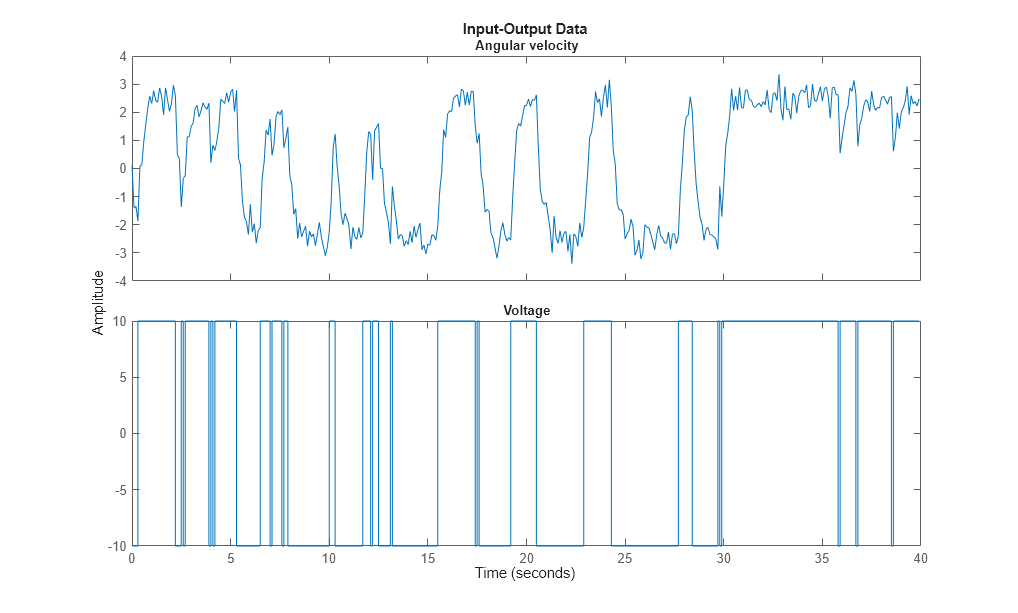Figure 2: Input-output data from a DC-motor.

Linear Modeling of the DC-Motor

1. Represent the DC motor structure in a function.

In this example, you use a MATLAB® file, but you can also use C MEX-files (to gain computational speed), P-files or function handles. For more information, see Creating IDNLGREY Model Files.

The DC-motor function is called `dcmotor_m.m` and is shown below.

` function [dx, y] = dcmotor_m(t, x, u, tau, k, varargin)`
``` % Output equations. y = [x(1); ... % Angular position. x(2) ... % Angular velocity. ];```
``` % State equations. dx = [x(2); ... % Angular velocity. -(1/tau)*x(2)+(k/tau)*u(1) ... % Angular acceleration. ];```

The file must always be structured to return the following:

Output arguments:

• `dx` is the vector of state derivatives in continuous-time case, and state update values in the discrete-time case.

• `y` is the output equation

Input arguments:

• The first three input arguments must be: `t` (time), `x` (state vector, [] for static systems), `u` (input vector, [] for time-series).

• Ordered list of parameters follow. The parameters can be scalars, column vectors, or 2-dimensional matrices.

• `varargin` for the auxiliary input arguments

2. Represent the DC motor dynamics using an `idnlgrey` object.

The model describes how the inputs generate the outputs using the state equation(s).

```FileName = 'dcmotor_m'; % File describing the model structure. Order = [2 1 2]; % Model orders [ny nu nx]. Parameters = [1; 0.28]; % Initial parameters. Np = 2. InitialStates = [0; 0]; % Initial initial states. Ts = 0; % Time-continuous system. nlgr = idnlgrey(FileName, Order, Parameters, InitialStates, Ts, ... 'Name', 'DC-motor'); ```

In practice, there are disturbances that affect the outputs. An `idnlgrey` model does not explicitly model the disturbances, but assumes that these are just added to the output(s). Thus, `idnlgrey` models are equivalent to Output-Error (OE) models. Without a noise model, past outputs do not influence prediction of future outputs, which means that predicted output for any prediction horizon k coincide with simulated outputs.

3. Specify input and output names, and units.

```set(nlgr, 'InputName', 'Voltage', 'InputUnit', 'V', ... 'OutputName', {'Angular position', 'Angular velocity'}, ... 'OutputUnit', {'rad', 'rad/s'}, ... 'TimeUnit', 's'); ```

4. Specify names and units of the initial states and parameters.

```nlgr = setinit(nlgr, 'Name', {'Angular position' 'Angular velocity'}); nlgr = setinit(nlgr, 'Unit', {'rad' 'rad/s'}); nlgr = setpar(nlgr, 'Name', {'Time-constant' 'Static gain'}); nlgr = setpar(nlgr, 'Unit', {'s' 'rad/(V*s)'}); ```

You can also use `setinit` and `setpar` to assign values, minima, maxima, and estimation status for all initial states or parameters simultaneously.

5. View the initial model.

a. Get basic information about the model.

The DC-motor has 2 (initial) states and 2 model parameters.

```size(nlgr) ```
```Nonlinear grey-box model with 2 outputs, 1 inputs, 2 states and 2 parameters (2 free). ```

b. View the initial states and parameters.

Both the initial states and parameters are structure arrays. The fields specify the properties of an individual initial state or parameter. Type `help idnlgrey.InitialStates` and `help idnlgrey.Parameters` for more information.

```nlgr.InitialStates(1) nlgr.Parameters(2) ```
```ans = struct with fields: Name: 'Angular position' Unit: 'rad' Value: 0 Minimum: -Inf Maximum: Inf Fixed: 1 ans = struct with fields: Name: 'Static gain' Unit: 'rad/(V*s)' Value: 0.2800 Minimum: -Inf Maximum: Inf Fixed: 0 ```

c. Retrieve information for all initial states or model parameters in one call.

For example, obtain information on initial states that are fixed (not estimated) and the minima of all model parameters.

```getinit(nlgr, 'Fixed') getpar(nlgr, 'Min') ```
```ans = 2x1 cell array {} {} ans = 2x1 cell array {[-Inf]} {[-Inf]} ```

d. Obtain basic information about the object:

```nlgr ```
```nlgr = Continuous-time nonlinear grey-box model defined by 'dcmotor_m' (MATLAB file): dx/dt = F(t, u(t), x(t), p1, p2) y(t) = H(t, u(t), x(t), p1, p2) + e(t) with 1 input(s), 2 state(s), 2 output(s), and 2 free parameter(s) (out of 2). Name: DC-motor Status: Created by direct construction or transformation. Not estimated. ```

Use `get` to obtain more information about the model properties. The `idnlgrey` object shares many properties of parametric linear model objects.

```get(nlgr) ```
``` FileName: 'dcmotor_m' Order: [1x1 struct] Parameters: [2x1 struct] InitialStates: [2x1 struct] FileArgument: {} SimulationOptions: [1x1 struct] Report: [1x1 idresults.nlgreyest] TimeVariable: 't' NoiseVariance: [2x2 double] Ts: 0 TimeUnit: 'seconds' InputName: {'Voltage'} InputUnit: {'V'} InputGroup: [1x1 struct] OutputName: {2x1 cell} OutputUnit: {2x1 cell} OutputGroup: [1x1 struct] Notes: [0x1 string] UserData: [] Name: 'DC-motor' ```

Performance Evaluation of the Initial DC-Motor Model

Before estimating the parameters `tau` and `k`, simulate the output of the system with the parameter guesses using the default differential equation solver (a Runge-Kutta 45 solver with adaptive step length adjustment). The simulation options are specified using the "SimulationOptions" model property.

1. Set the absolute and relative error tolerances to small values (`1e-6` and `1e-5`, respectively).

```nlgr.SimulationOptions.AbsTol = 1e-6; nlgr.SimulationOptions.RelTol = 1e-5; ```

2. Compare the simulated output with the measured data.

`compare` displays both measured and simulated outputs of one or more models, whereas `predict`, called with the same input arguments, displays the simulated outputs.

The simulated and measured outputs are shown in a plot window.

```compare(z, nlgr); ```Figure 3: Comparison between measured outputs and the simulated outputs of the initial DC-motor model.

Parameter Estimation

Estimate the parameters and initial states using `nlgreyest`, which is a prediction error minimization method for nonlinear grey box models. The estimation options, such as the choice of estimation progress display, are specified using the "nlgreyestOptions" option set.

```nlgr = setinit(nlgr, 'Fixed', {false false}); % Estimate the initial states. opt = nlgreyestOptions('Display', 'on'); nlgr = nlgreyest(z, nlgr, opt); ```

Performance Evaluation of the Estimated DC-Motor Model

1. Review the information about the estimation process.

This information is stored in the `Report` property of the `idnlgrey` object. The property also contains information about how the model was estimated, such as solver and search method, data set, and why the estimation was terminated.

```nlgr.Report fprintf('\n\nThe search termination condition:\n') nlgr.Report.Termination ```
```ans = Status: 'Estimated using NLGREYEST' Method: 'Solver: ode45; Search: lsqnonlin' Fit: [1x1 struct] Parameters: [1x1 struct] OptionsUsed: [1x1 idoptions.nlgreyest] RandState: [] DataUsed: [1x1 struct] Termination: [1x1 struct] The search termination condition: ans = struct with fields: WhyStop: 'Change in cost was less than the specified tolerance.' Iterations: 5 FirstOrderOptimality: 1.4013e-04 FcnCount: 6 Algorithm: 'trust-region-reflective' ```

2. Evaluate the model quality by comparing simulated and measured outputs.

The fits are 98% and 84%, which indicate that the estimated model captures the dynamics of the DC motor well.

```compare(z, nlgr); ```Figure 4: Comparison between measured outputs and the simulated outputs of the estimated IDNLGREY DC-motor model.

3. Compare the performance of the `idnlgrey` model with a second-order ARX model.

```na = [2 2; 2 2]; nb = [2; 2]; nk = [1; 1]; dcarx = arx(z, [na nb nk]); compare(z, nlgr, dcarx); ```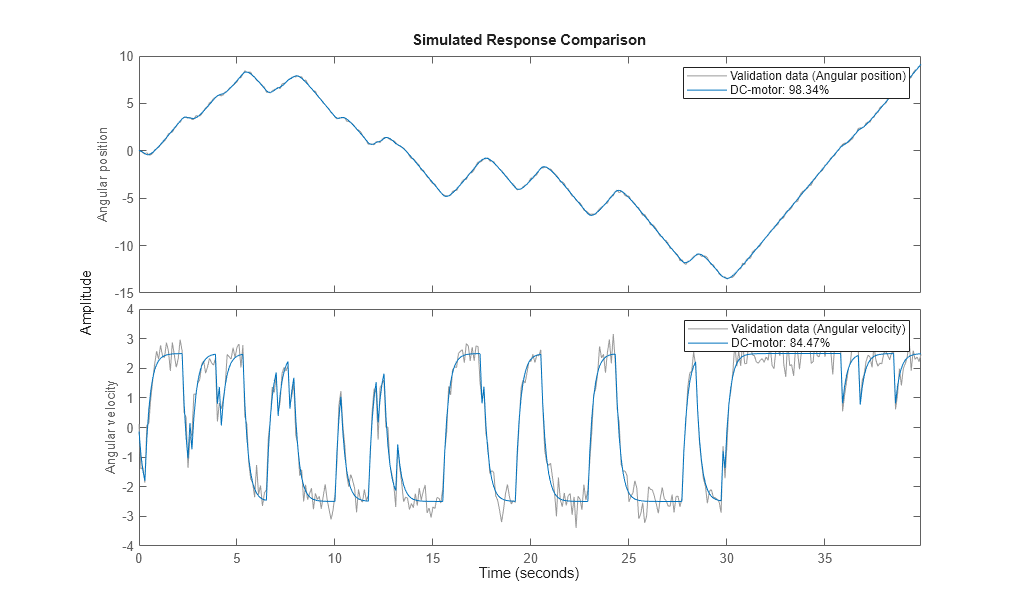Figure 5: Comparison between measured outputs and the simulated outputs of the estimated IDNLGREY and ARX DC-motor models.

4. Check the prediction errors.

The prediction errors obtained are small and are centered around zero (non-biased).

```pe(z, nlgr); ```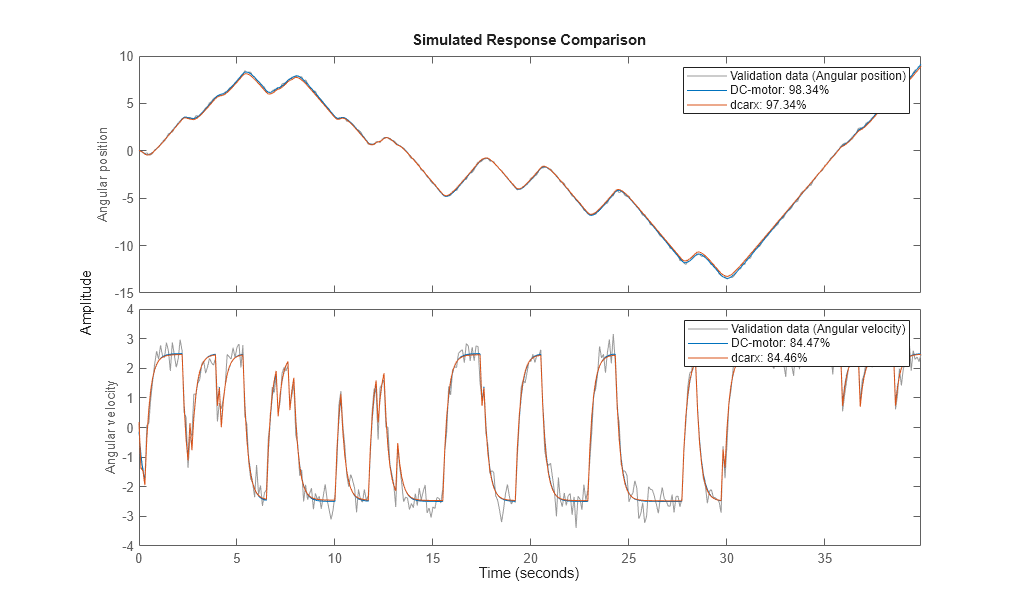Figure 6: Prediction errors obtained with the estimated IDNLGREY DC-motor model.

5. Check the residuals ("leftovers").

Residuals indicate what is left unexplained by the model and are small for good model quality. Use the `resid` command to view the correlations among the residuals. The first column of plots shows the autocorrelations of the residuals for the two outputs. The second column shows the cross-correlation of these residuals with the input "Voltage". The correlations are within acceptable bounds (blue region).

```figure('Name',[nlgr.Name ': residuals of estimated model']); resid(z,nlgr); ```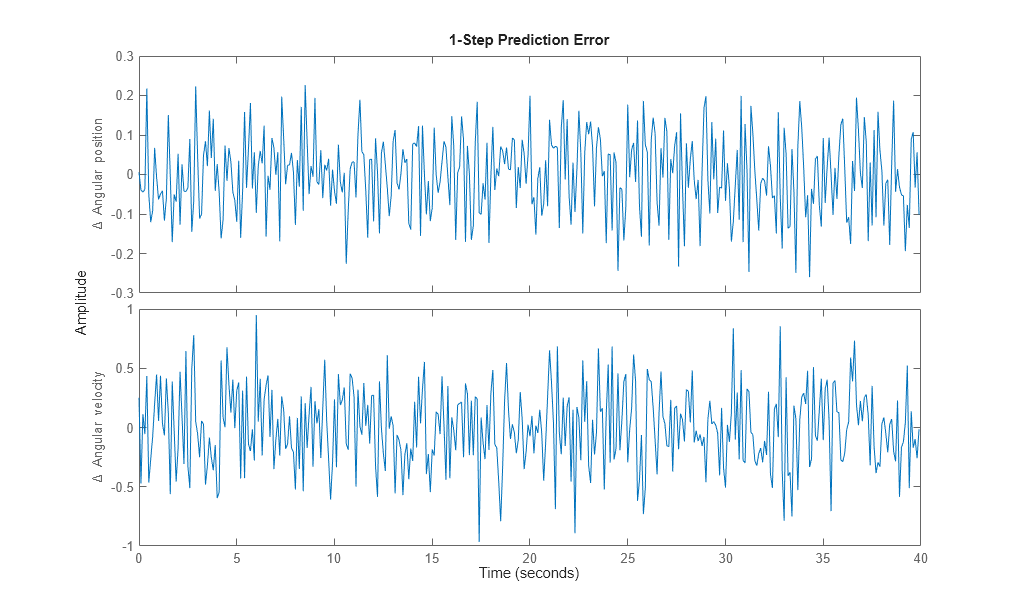Figure 7: Residuals obtained with the estimated IDNLGREY DC-motor model.

6. Plot the step response.

A unit input step results in an angular position showing a ramp-type behavior and to an angular velocity that stabilizes at a constant level.

```figure('Name', [nlgr.Name ': step response of estimated model']); step(nlgr); ```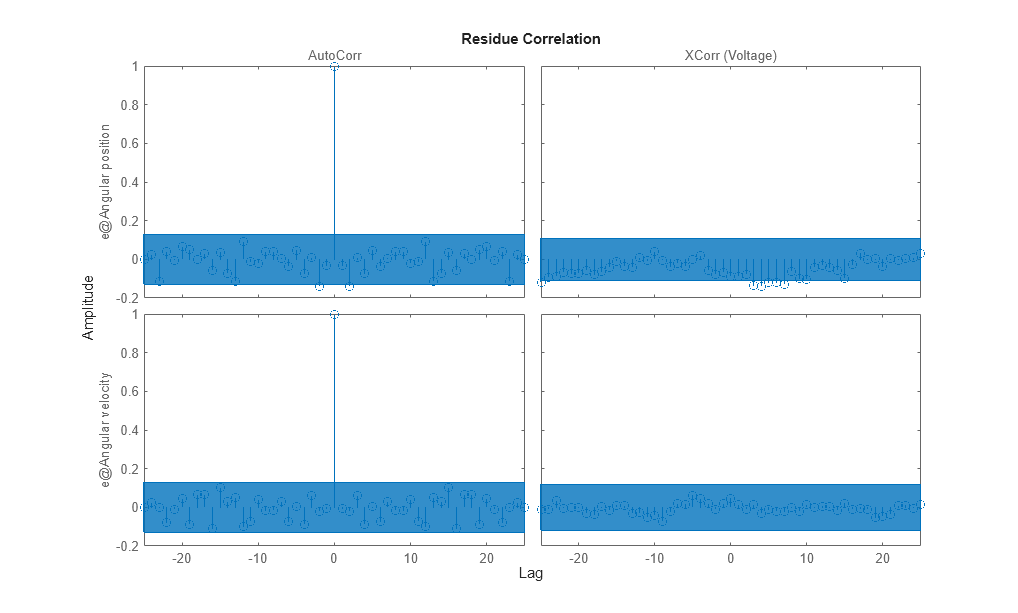Figure 8: Step response with the estimated IDNLGREY DC-motor model.

7. Examine the model covariance.

You can assess the quality of the estimated model to some extent by looking at the estimated covariance matrix and the estimated noise variance. A "small" value of the `(i, i)` diagonal element of the covariance matrix indicates that the `i`:th model parameter is important for explaining the system dynamics when using the chosen model structure. Small noise variance (covariance for multi-output systems) elements are also a good indication that the model captures the estimation data in a good way.

```getcov(nlgr) nlgr.NoiseVariance ```
```ans = 1.0e-04 * 0.1573 0.0021 0.0021 0.0008 ans = 0.0010 -0.0000 -0.0000 0.0110 ```

For more information about the estimated model, use `present` to display the initial states and estimated parameter values, and estimated uncertainty (standard deviation) for the parameters.

```present(nlgr); ```
``` nlgr = Continuous-time nonlinear grey-box model defined by 'dcmotor_m' (MATLAB file): dx/dt = F(t, u(t), x(t), p1, p2) y(t) = H(t, u(t), x(t), p1, p2) + e(t) with 1 input(s), 2 state(s), 2 output(s), and 2 free parameter(s) (out of 2). Inputs: u(1) Voltage(t) [V] States: Initial value x(1) Angular position(t) [rad] xinit@exp1 0.0302675 (estimated) in [-Inf, Inf] x(2) Angular velocity(t) [rad/s] xinit@exp1 -0.133777 (estimated) in [-Inf, Inf] Outputs: y(1) Angular position(t) [rad] y(2) Angular velocity(t) [rad/s] Parameters: Value Standard Deviation p1 Time-constant [s] 0.243649 0.00396671 (estimated) in [-Inf, Inf] p2 Static gain [rad/(V*s)] 0.249644 0.000284486 (estimated) in [-Inf, Inf] Name: DC-motor Status: Termination condition: Change in cost was less than the specified tolerance.. Number of iterations: 5, Number of function evaluations: 6 Estimated using Solver: ode45; Search: lsqnonlin on time domain data "DC-motor". Fit to estimation data: [98.34;84.47]% FPE: 0.001096, MSE: 0.1187 More information in model's "Report" property. ```

Conclusions

This example illustrates the basic tools for performing nonlinear grey-box modeling. See the other nonlinear grey-box examples to learn about:

• Using nonlinear grey-box models in more advanced modeling situations, such as building nonlinear continuous- and discrete-time, time-series and static models.

• Writing and using C MEX model-files.

• Handling nonscalar parameters.

• Impact of certain algorithm choices.

For more information on identification of dynamic systems with System Identification Toolbox, visit the System Identification Toolbox product information page.

### Nonlinear Grey-Box Model Properties and Estimation Options

`idnlgrey` creates a nonlinear grey-box model based on the model structure and properties. The parameters and initial states of the created `idnlgrey` object are estimated using `nlgreyest`.

The following model properties and estimation options affect the model creation and estimation results.

#### Simulation Method

You specify the simulation method using the `SimulationOptions` (`struct`) property of `idnlgrey`.

System Identification Toolbox™ software provides several variable-step and fixed-step solvers for simulating `idnlgrey` models.

For discrete-time systems, the default solver is `'FixedStepDiscrete'`. For continuous-time systems, the default solver is `'ode45'`. By default, `SimulationOptions.Solver` is set to `'Auto'`, which automatically selects either `'ode45'` or `'FixedStepDiscrete'` during estimation and simulation—depending on whether the system is continuous or discrete in time.

To view a list of available solvers and their properties, see the `SimulationOptions` model property in `idnlgrey` reference page.

#### Search Method

You specify the search method for estimating model parameters using the `SearchMethod` option of the `nlgreyestOptions` option set. Two categories of methods are available for nonlinear grey-box modeling.

One category of methods consists of the minimization schemes that are based on line-search methods, including Gauss-Newton type methods, steepest-descent methods, and Levenberg-Marquardt methods.

The Trust-Region Reflective Newton method of nonlinear least-squares (`lsqnonlin`), where the cost is the sum of squares of errors between the measured and simulated outputs, requires Optimization Toolbox™ software. When the parameter bounds differ from the default +/- Inf, this search method handles the bounds better than the schemes based on a line search. However, unlike the line-search-based methods, `lsqnonlin` cannot handle automatic weighting by the inverse of estimated noise variance in multi-output cases. For more information, see `OutputWeight` estimation option in the `nlgreyestOptions` reference page.

By default, `SearchMethod` is set to `Auto`, which automatically selects a method from the available minimizers. If the Optimization Toolbox product is installed, `SearchMethod` is set to `'lsqnonlin'`. Otherwise, `SearchMethod` is a combination of line-search based schemes.

For detailed information about this and other `nlgreyest` estimation options, see `nlgreyestOptions`.

You specify the method for calculating gradients using the `GradientOptions` option of the `nlgreyestOptions` option set. Gradients are the derivatives of errors with respect to unknown parameters and initial states.

Gradients are calculated by numerically perturbing unknown quantities and measuring their effects on the simulation error.

Option for gradient computation include the choice of the differencing scheme (forward, backward or central), the size of minimum perturbation of the unknown quantities, and whether the gradients are calculated simultaneously or individually.

For detailed information about this and other `nlgreyest` estimation options, see `nlgreyestOptions`.